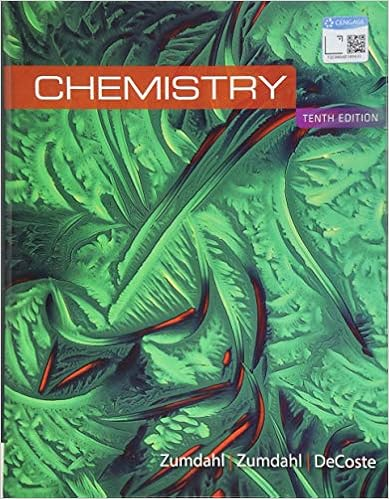# Solution a the balanced chemical equations are

• Notes
• MCQubed
• 74
• 100% (3) 3 out of 3 people found this document helpful

This preview shows page 62 - 64 out of 74 pages.

##### We have textbook solutions for you!
The document you are viewing contains questions related to this textbook.The document you are viewing contains questions related to this textbook.
Chapter 17 / Exercise 35
Chemistry
ZumdahlExpert Verified
Solution: a) The balanced chemical equations are: Methane: CH 4 ( g ) + 2O 2 ( g ) CO 2 ( g ) + 2H 2 O( l ) Propane: C 3 H 8 ( g ) + 5O 2 ( g ) 3CO 2 ( g ) + 4H 2 O( l ) Mass (g) of CO 2 from each: Methane: 4 2 2 4 4 2 1 mol CH 1 mol CO 44.01 g CO 25.0% 200.g Mixture 100% 16.04 g CH 1 mol CH 1 mol CO       = 137.188 g CO 2 Propane: 3 8 2 2 3 8 3 8 2 1 mol C H 3 mol CO 44.01 g CO 75.0% 200.g Mixture 100% 44.09 g C H 1 mol C H 1 mol CO      = 449.183 g CO 2 Total CO 2 = 137.188 g + 449.183 g = 586.371 = 586 g CO 2 b) Since the mass of CH 4 + the mass of C 3 H 8 = 252 g, let x = mass of CH 4 in the mixture and 252 – x = mass of C 3 H 8 in the mixture. Use mole ratios to calculate the amount of CO 2 formed from x amount of CH 4 and the amount of CO 2 formed from 252 – x amount of C 3 H 8 . The total mass of CO 2 produced = 748 g. The total moles of CO 2 produced = 2 2 2 1 mol CO 748 g CO 44.01 g CO = 16.996 mol CO 2 16.996 mol CO 2 = 3 8 4 2 2 4 3 8 4 4 3 8 3 8 1 mol C H 1 mol CH 1 mol CO 3 mol CO x g CH + 252 x g C H 16.04 g CH 1 mol CH 44.09 g C H 1 mol C H      
##### We have textbook solutions for you!
The document you are viewing contains questions related to this textbook.The document you are viewing contains questions related to this textbook.
Chapter 17 / Exercise 35
Chemistry
ZumdahlExpert Verified
3-63 16.996 mol CO 2 = 2 2 x 3(252 x) mol CO + mol CO 16.04 44.09 16.996 mol CO 2 = 2 2 x 756 3x mol CO + mol CO 16.04 44.09 16.996 mol CO 2 = 0.06234x mol CO 2 + (17.147 – 0.06804x mol CO 2 ) 16.996 = 17.147 – 0.0057x x = 26.49 g CH 4 252 – x = 252 g – 26.49 g = 225.51 g C 3 H 8 Mass % CH 4 = 4 4 mass of CH 26.49 g CH 100 = 100 mass of mixture 252 g mixture = 10.5% CH 4 Mass % C 3 H 8 = 3 8 3 8 mass of C H 225.51 g C H 100 = 100 mass of mixture 252 g mixture = 89.5% C 3 H 8 3.141 Plan: Find the number of moles of HCl in the final solution and the number of moles of HCl in the solution whose molarity is known. Subtract the moles of HCl in the known solution from the number of moles of HCl in the final solution to find the moles of HCl in the solution of unknown molarity. Divide that number of moles of HCl by the volume of that solution to find its molarity. Solution: Total volume (L) of the final solution = 1.35 L + 3.57 L = 4.92 L Total moles of HCl in final solution = 0.893 mol HCl 4.92 L 1 L = 4.39356 mol HCl Moles of HCl in solution of known concentration = 0.325 mol HCl 1.35 L 1 L = 0.43875 mol HCl Moles of HCl added from solution with unknown concentration = Total moles moles from known solution = (4.39356 0.43875) mol HCl = 3.95481 mol HCl Molarity of unknown solution = moles solute 3.95481 mol HCl = L of solution 3.57 L = 1.10779 = 1.11 M HCl 3.142 Plan: If we assume a 100-gram sample of fertilizer, then the 30:10:10 percentages become the masses, in grams, of N, P 2 O 5 , and K 2 O. These masses may be changed to moles of substance, and then to moles of each element. To get the desired x:y:1.0 ratio, divide the moles of each element by the moles of potassium.
•••